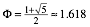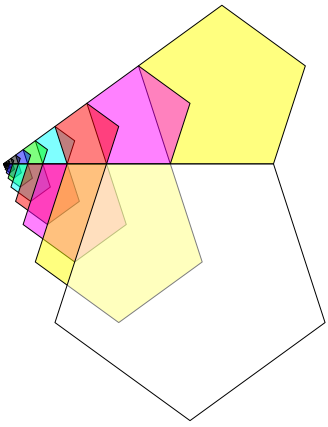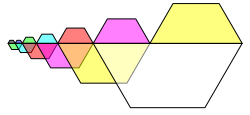Hans Walser, 

Visualizations of the Fibonacci recursion

Figure 1 depicts a classical visualization.Fig. 1: Classical

We have a spiral arrangement without overlapping squares.

In this figure and also in the following we use a color code according to table 1.

 No RGB Color Example New No Fibonacci 0 0,0,0 Black 1 1 1 0,0,1 Blue 2 1 2 0,1,0 Green 3 2 3 0,1,1 Cyan 4 3 4 1,0,0 Red 5 5 5 1,0,1 Magenta 6 8 6 1,1,0 Yellow 7 13 7 1,1,1 White 8 21

Tab. 1: Color code

In figure 2 we have a linear arrangement.Fig. 2: Linear arrangement

This can be done also with other polygons. The figure 3 gives a version with regular triangles.Fig. 3: Regular triangles

The figure 4 works with regular pentagons.Fig. 4: Regular pentagons

The “roofline” is not straightforward, but interrupted.

Taking a geometric sequence based on the golden sectionleads to a proper roofline (Fig. 5). But this is no more a Fibonacci sequence. The difference is visible at the beginning.Fig. 5: Golden section

Parts of this figure can be used to draw a regular pentagon (Fig. 6).Fig. 6: Pentagon

In the following figures we will work again with the Fibonacci sequence.

The figure 7 uses half hexagons.Fig. 7: Half hexagons

In figure 8 half circles.Fig. 8: Half circles

In figure 9 half circles again, but this time with a golden geometric sequence.Fig. 9: Golden section